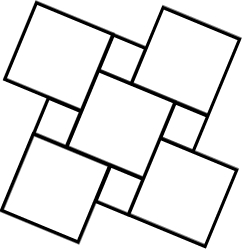# Right-angled 64614

Arrange the given shapes according to their area content, in descending order:
Square with perimeter = 16 cm
A rectangle with side a = 3 cm and perimeter o = 16 cm
A right-angled triangle with a hypotenuse of 4.125 cm and a hypotenuse of 8.125 cm

x = TOS

### Step-by-step explanation:Did you find an error or inaccuracy? Feel free to write us. Thank you!

Tips for related online calculators
Need help calculating sum, simplifying, or multiplying fractions? Try our fraction calculator.